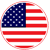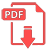# Home | Mathematics | Geometry(Planimetry)

## Circles

A circle is all points in the same plane that lie at an equal distance from a center point. The circle is only composed of the points on the border. You could think of a circle as a hula hoop. It's only the points on the border that are the circle. The points within the hula hoop are not part of the circle and are called interior points.

The distance between the midpoint and the circle border is called the radius. A line segment that has the endpoints on the circle and passes through the midpoint is called the diameter. The diameter is twice the size of the radius. A line segment that has its endpoints on the circular border but does not pass through the midpoint is called a chord.The distance around the circle is called the circumference, C, and could be determined either by using the radius, r, or the diameter, d:

C=2πr

C=πdEnglish